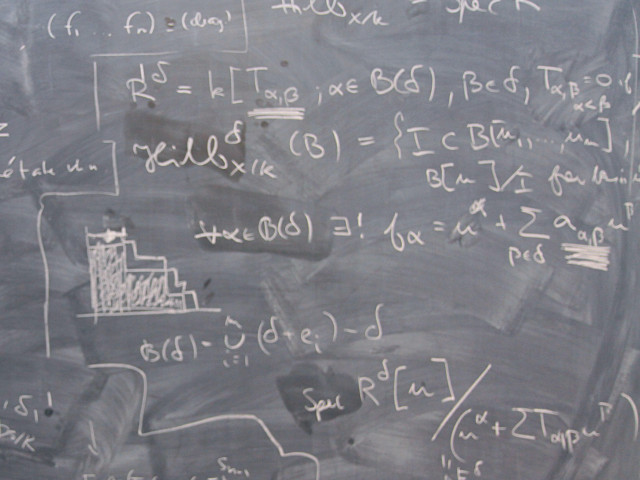# SF2832 Mathematical Systems Theory 7.5 credits

#### Matematisk systemteori### Offering and execution

#### No offering selected

Select the semester and course offering above to get information from the correct course syllabus and course offering.

## Course information

### Content and learning outcomes

#### Course contents *

Linear control systems: reachability, observability, stability, realization theory, minimality, feedback, pole-assignment, observers. Linear-quadratic optimal control, matrix Riccati equation and theory for the algebraic Riccati equation. Kalman filtering.

#### Intended learning outcomes *

This is an introductory course in mathematical systems theory. The subject provides the mathematical foundation of modern control theory, with application in aeronautics, electrical networks, signal processing, and many other areas. The aim of the course is that you should acquire a systematic understanding of linear dynamical systems, which is the focus of this course. The acquirement of such knowledge is not only very useful preparation for work on system analysis and design problems that appear in many engineering fields, but is also necessary for further studies in control and signal processing.

The overall goal of the course is to provide an understanding of the basic ingredients of linear systems theory and how these are used in analysis and design of control, estimation and filtering systems. In the course we take the state-space approach, which is well suited for efficient control and estimation design.

Measurable goals:

To pass the course, the student should be able to do the following:

• Analyze the state-space model with respect to minimality, observability, reachability, detectability and stabilizability.
• Explain the relationship between input-output (external) models and state-space (internal) models for linear systems and derive such models from the basic principles.
• Derive a minimal state-space model using the Kalman decomposition.
• Use algebraic design methods for state feedback design with pole assignment, and construct stable state observers by pole assignment and analyze the properties of the closed loop system obtained when the observer and the state feedback are combined to an observer based controller.
• Apply linear quadratic techniques to derive optimal state feedback controllers.
• Design a Kalman filter for optimal state estimation of linear systems subject to stochastic disturbances.
• Solve the Riccati equations that appear in optimal control and estimation problems.
• Apply the methods given in the course to solve example problems (one should also be able to use the Control System Toolbox in Matlab to solve the linear algebra problems that appear in the examples).

• Integrate the tools learnt during the course and apply them to more complex problems.
• Explain how the above results and methods relate and build on each other.
• Explain the mathematical (mainly linear algebra) foundations of the techniques used in linear systems theory and apply those techniques flexibly to variations of the problems studied in the course.
• Solve fairly simple but realistic control design problems using the methods in the course.

#### Course Disposition

No information inserted

### Literature and preparations

#### Specific prerequisites *

In general:

150 university credits (hp) including 28 hp in Mathematics and 6 hp in Mathematical Statistics. Documented proficiency in English corresponding to English B.

More precisely for KTH students:

Passed courses in calculus, linear algebra, differential equations, mathematical statistics, numerical analysis. A passed course in control theory is an advantage.

#### Recommended prerequisites

The prerequisites is a Swedish or foreign degree equivalent to Bachelor of Science of 180 ECTS credits, with at least 45 ECTS credits in mathematics. The students should have documented knowledge corresponding to basic university courses in analysis, linear algebra, numerical analysis, differential equations and transforms, mathematical statistics, and optimization.

#### Equipment

No information inserted

#### Literature

Lindquist & Sand: An introduction to mathematical systems theory.

Material from the department of Mathematics.

### Examination and completion

A, B, C, D, E, FX, F

#### Examination *

• TENA - Examination, 7.5 credits, Grading scale: A, B, C, D, E, FX, F

Based on recommendation from KTH’s coordinator for disabilities, the examiner will decide how to adapt an examination for students with documented disability.

The examiner may apply another examination format when re-examining individual students.

#### Other requirements for final grade *

A written examination (TENA; 7,5 hp).
Optional homework sets that give bonus credit on the exam.

#### Opportunity to complete the requirements via supplementary examination

No information inserted

#### Opportunity to raise an approved grade via renewed examination

No information inserted

### Further information

#### Course web

Further information about the course can be found on the Course web at the link below. Information on the Course web will later be moved to this site.

Course web SF2832

SCI/Mathematics

Mathematics

Second cycle